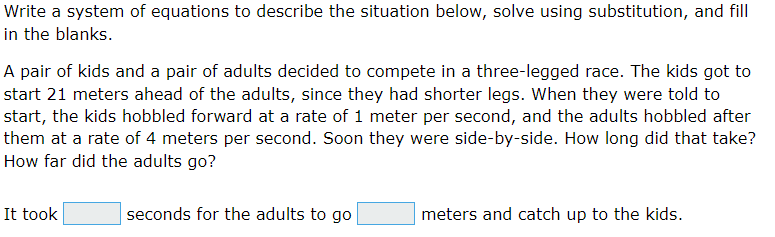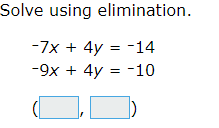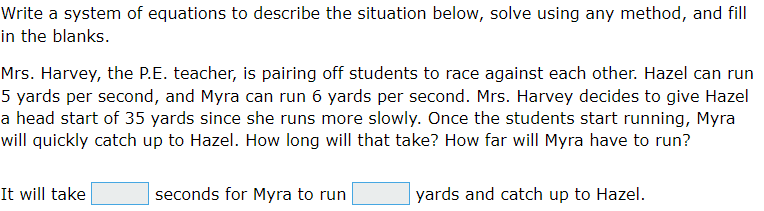# Solving System of Linear Equations

This set of MCQs helps you brush up on important math topics and prepare you to dive into skill practice.

Start Quiz

Which of the following equations are linear equations in two variables?

6/y​ + 4 = -2x

6/y​ + 4 = 0

5a^3–4=4b

5a^3=4

Form a linear equation in two variables for the following situation: "The sum of two numbers is 186."

j×k=186

j+k=186

j-k=186

j/k=186

Which one of the following options is true, y = 3x + 5 has.

Two solutions

Only one solutions

Infinitely many solutions

No solutionsIt took 28 seconds for the adults to go 7 meters and catch up to the kids.

It took 6 seconds for the adults to go 28 meters and catch up to the kids.

It took 7 seconds for the adults to go 28 meters and catch up to the kids.

It took 6 seconds for the adults to go 38 meters and catch up to the kids.(–2, –2)

(–2, –7)

(–2, 7)

(2, –7)

Solve for y.

7x + y = –12
x = –3

-9

9

33

31It will take 35 seconds for Myra to run 210 yards and catch up to Hazel.

It will take 25 seconds for Myra to run 210 yards and catch up to Hazel.

It will take 353 seconds for Myra to run 210 yards and catch up to Hazel.

It will take 35 seconds for Myra to run 230 yards and catch up to Hazel.

Solve for v.
10v = 10

1/2

0

-1

1

Quiz/Test Summary
Title: Solving System of Linear Equations
Questions: 8
Contributed by: